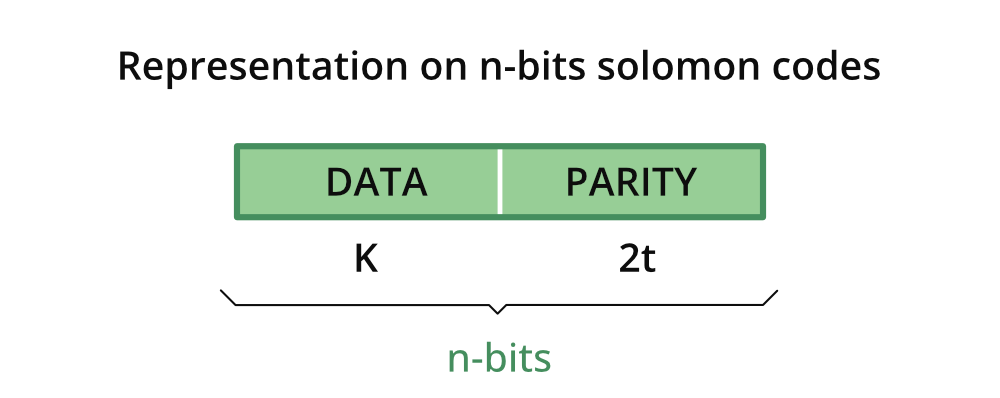Open in App
Not now

# What is Reed–Solomon Code?

• Last Updated : 24 Feb, 2022

When we transfer some data over a network then there are possibilities that data get corrupted due to some network problem. Bits inside data might get corrupted due to interference or some network problem. So there is the risk of data being lost during transfer.

### Reed-Solomon codes

Reed-Solomon codes are the code that was introduced by Irving S. Reed and Gustave Solomon. Reed-Solomon code is a subclass of non-binary BCH codes. The encoder of Reed-Solomon codes differs from a binary encoder in that it operates on multiple bits rather than individual bits.

So basically, Reed-Solomon codes help in recovering corrupted messages that are being transferred over a network. In Reed-Solomon codes, we have:

• Encoder and
• Decoder

Reed-Solomon codes encoder receives data and before transferring it over the noisy network it adds some parity bits with our original data bits.

On the other hand, we have a Reed-Solomon codes decoder that detects corrupted messages and recovers them from error.

### Representation of n-bits Reed-Solomon codesn-bits representation of the Reed-Solomon codes

### Parameters of Reed-Solomon code:

• (n, k) code is used to encode m-bit symbols.
• Block length(n) is given by 2m-1 symbols.
• In Reed-Solomon codes, message size is given by (n-2t) where t= number of errors corrected.
• Parity check size is given by = (n-k) or 2t symbols.
• Minimum distance(a) is given by = (2t+1).
• Message size is of k bits.

### Generator function

In Reed-Solomon codes, the generator function is generated using a special polynomial. In Reed-Solomon codes, all valid codewords are exactly divisible by the generator polynomial. The generator function is given by:

g(x) = (x-α)(x-α2)(x-α3)……(x-α2t)

### Encoding

We perform encoding in Reed-Solomon codes with the following methods:

• Consider a Reed-Solomon code with parameters n(block size), k(message size), q(symbol size in bits). For encoding, we encode the message as a polynomial p(x) and then multiply it with a code generator polynomial g(x)
where g(x) = (x-α)(x-α2)(x-α3)……(x-α2t)
• Then we map the message vector[x1,x2,…..,xk] to a polynomial p(x) of degree<k such that
pxi) = xi for all i=1,2,3,….k
• Polynomial can be done using Lagrange interpolation.
• Sender calculates s(x) = p(x)*g(x) and then sends over the coefficients of s(x)

### Decoding

At the receiver end we perform the following methods:

• If s(x)== r(x) then r(x)/g(x) has no remainder.
• If it has remainder, then r(x) = p(x) * g(x) + e(x) where e(x) is an error polynomial.

### Application of Reed-Solomon codes

• It is used in storage devices like CDs, DVDs, etc.
• It is used in wireless or mobile communication for data transfer.
• It is used in satellite communication.
• Reed-Solomon codes are also used in digital TV.
• It is used in high-speed modems.
• It is used in the BAR code, QR code.

Here we will discuss how it is better than binary BCH codes.

• It has the highest efficient use of redundancy.
• It is possible to adjust block length and symbol size in Reed-Solomon codes.
• It provides a wide range of code rates.
• In Reed-Solomon codes, there are efficient decoding techniques available.